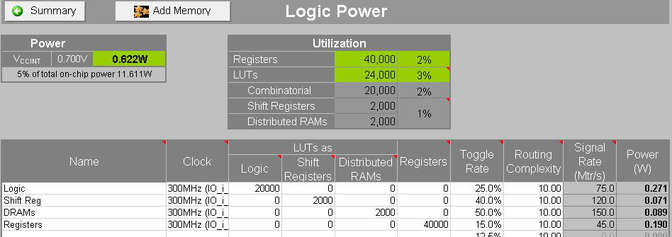# Logic - 2020.2 English

## Xilinx Power Estimator User Guide for Versal ACAP (UG1275)

Document ID
UG1275
Release Date
2020-12-04
Version
2020.2 English

The Logic sheet covers power estimates of CLB logic: LUTs and Registers as shown in the following figure. Each row represents a group of logic that is associated with:

• A particular Clock whose frequency is used to calculate dynamic power.
• A Toggle Rate which represents an average over the inputs and outputs of all logic.

LUTs fall into the following three categories while Registers are primarily the CLB registers.

LUT as Logic
For simplified entry, XPE assumes an average sized LUT of about five inputs and also assumes a small percentage of LUTs use two outputs.
LUT as Shift Registers
SRL primitives.
LUT as Distributed RAMs
LUTRAM primitives.
Figure 1. XPE LogicBoth Shift Registers and Distributed RAMs use the M-type CLB LUTs that you can configure as memory. It may be difficult to estimate the total LUTs needed for Distributed RAM-based memories. Instead use the Add Memory button to launch the XPE Memory Configuration wizard. Specify the memory array size, clock and options and XPE calculates the expected number of LUTs and registers and enters them into a row. Toggle Rate is defined as the percentage of clock cycles where a transition occurs. The default value of 12.5% means one transition every eight cycles.

Routing Complexity is an abstract model of the interconnect power. The number represents the average number of routing resources per logical net. A design with higher complexity requires more routing resources per net which increases power. Routing Complexity is typically only modified when importing Vivado power analysis results where Routing Complexity is calculated from the actual routing resources used to route the design. For early estimation, Xilinx recommends that you leave Routing Complexity at the default setting.